# 1482 and Level 4

### Today’s Puzzle:

Can you write all the numbers from 1 to 10 in both the first column and the top row so that those numbers become the factors of the given clues?### Factors of 1482:

• 1482 is a composite number.
• Prime factorization: 1482 = 2 × 3 × 13 × 19
• 1482 has no exponents greater than 1 in its prime factorization, so √1482 cannot be simplified.
• The exponents in the prime factorization are 1, 1, 1, and 1. Adding one to each exponent and multiplying we get (1 + 1)(1 + 1)(1 + 1)(1 + 1) = 2 × 2 × 2 × 2 = 16. Therefore 1482 has exactly 16 factors.
• The factors of 1482 are outlined with their factor pair partners in the graphic below.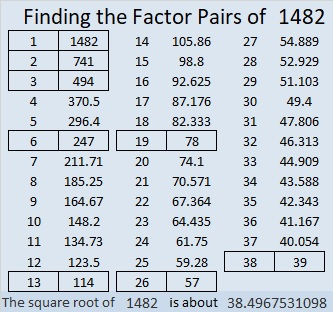### Another Fact about the Number 1482:

1482 is the hypotenuse of a Pythagorean triple:
570-1368-1482 which is (5-12-13) times 114.

# 1481 is the First Prime Number in the Fifth Prime Decade

### What Kind of Prime Number is 1481?

1481 is the 234th prime number. It is part of a twin prime pair, a prime triplet, a prime quadruplet, and even a prime quintuplet. All of those designations are nice, but to me, the most beautiful is the prime decade (the prime quadruplets not counting (5, 7, 11, 13)).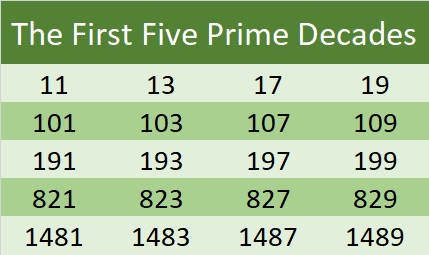1481 is the first prime number in the fifth prime decade. The last digits of the numbers in every prime decade make a lovely pattern: 1, 3, 7, and 9. Once you know the first prime number in the decade, you also know the other three! That’s beautiful!

I found the numbers in the first five prime decades easy to memorize. We start with the teen decade followed by the first and last decades in the 100’s. Then199 is almost 200 and that helps me remember 821, and 821 helps me remember 1481.

### Factors of 1481:

• 1481 is a prime number.
• Prime factorization: 1481 is prime.
• 1481 has no exponents greater than 1 in its prime factorization, so √1481 cannot be simplified.
• The exponent in the prime factorization is 1. Adding one to that exponent we get (1 + 1) = 2. Therefore 1481 has exactly 2 factors.
• The factors of 1481 are outlined with their factor pair partners in the graphic below.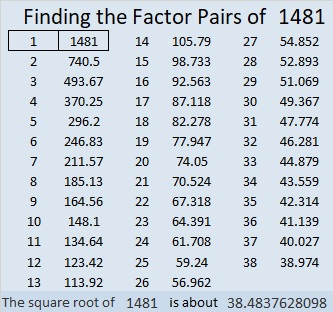How do we know that 1481 is a prime number? If 1481 were not a prime number, then it would be divisible by at least one prime number less than or equal to √1481. Since 1481 cannot be divided evenly by 2, 3, 5, 7, 11, 13, 17, 19, 23, 29 31, or 37, we know that 1481 is a prime number.

### More about the Number 1481:

1481 is the sum of two squares:
35² + 16² = 1481.

1481 is the hypotenuse of a Pythagorean triple:
969-1120-1481, calculated from 35² – 16², 2(35)(16), 35² + 16².

Here’s another way we know that 1481 is a prime number: Since its last two digits divided by 4 leave a remainder of 1, and 35² + 16² = 1481 with 35 and 16 having no common prime factors, 1481 will be prime unless it is divisible by a prime number Pythagorean triple hypotenuse less than or equal to √1481. Since 1481 is not divisible by 5, 13, 17, 29, or 37, we know that 1481 is a prime number.

# 1466 A Non-Simplified Yet Easy-to-Remember Unit Circle

### Look at the Patterns in this Unit Circle:

A unit circle has a radius of one and is often used to display the sines and cosines of special angles. I’ve made a unit circle that showcases the patterns that make memorizing how to complete one easier.

This unit circle is easy to memorize because many of the values have not been simplified. However, always remember to simplify the values when giving your final answer on any assignment or test!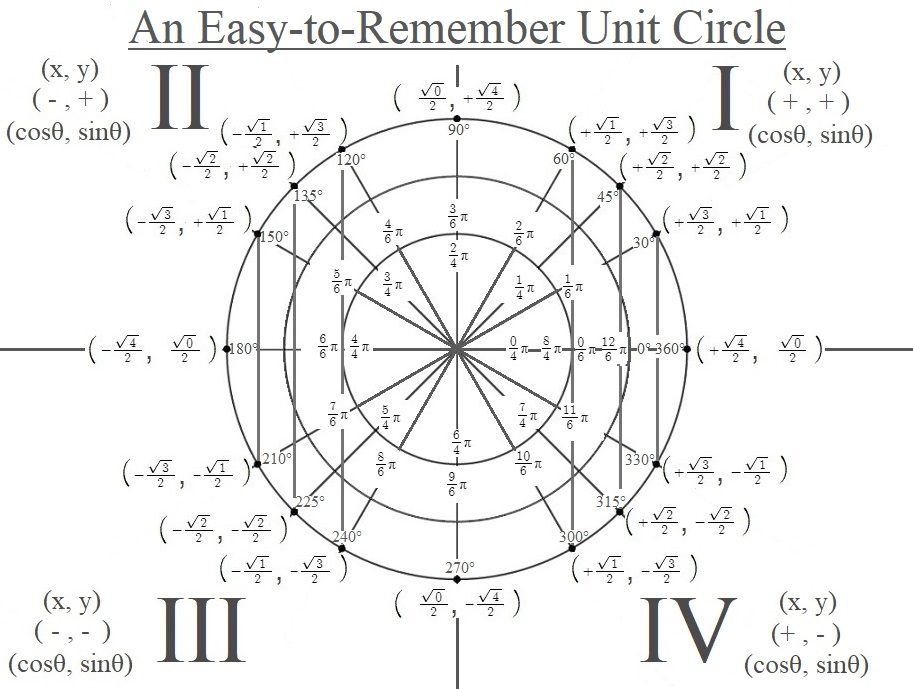The patterns you see in the ordered pairs representing the cosines and sines are only coincidental: they are merely an aid somebody else created to make memorizing the values easier. Over half of the square roots can be simplified and should be. However, I do love them in their non-simplified form because it is easy to remember them, and it is easy to see the sine wave and the cosine wave as the square root values increase and decrease incrementally as we go around the unit circle.

I’ve also made a similar unit circle that might help students locate the angles in the quadrants better: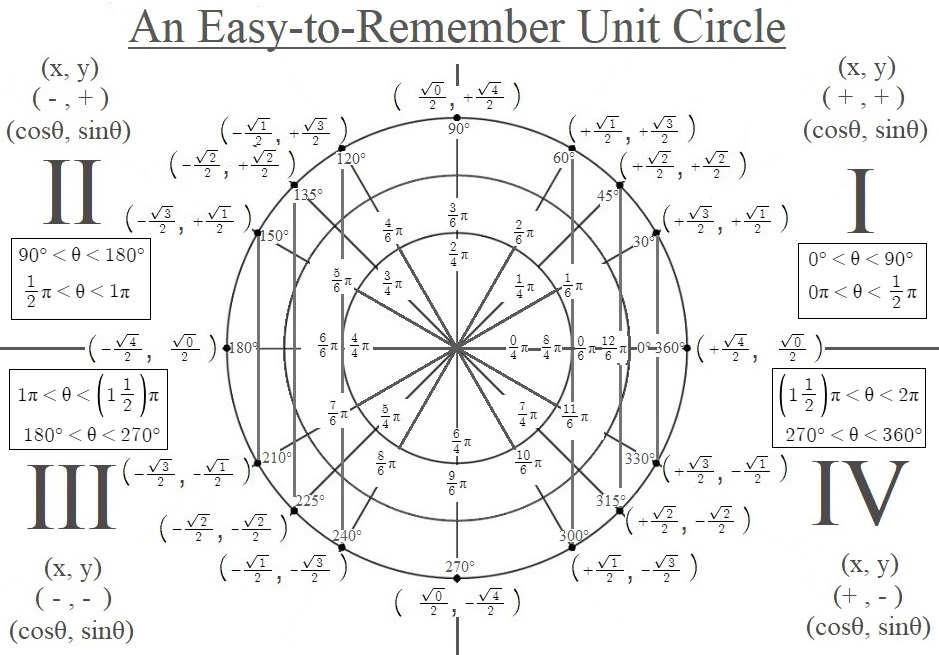### Factors of 1466:

• 1466 is a composite number.
• Prime factorization: 1466 = 2 × 733
• 1466 has no exponents greater than 1 in its prime factorization, so √1466 cannot be simplified.
• The exponents in the prime factorization are 1, and 1. Adding one to each exponent and multiplying we get (1 + 1)(1 + 1) = 2 × 2 = 4. Therefore 1466 has exactly 4 factors.
• The factors of 1466 are outlined with their factor pair partners in the graphic below.### More Facts about the number 1466:

1466 is the sum of two squares:
29² + 25² = 1466

1466 is also the hypotenuse of a Pythagorean triple:
216-1450-1466 calculated from 29² – 25², 2(29)(25), 29² + 25²

# 1465 A Degrees to and from Radians Calculator

Elementary and middle school students measure their angles in degrees. When they get to high school, they find out that sometimes we prefer to measure angles in radians. Of course, they also need to convert degrees to radians and to convert radians into degrees.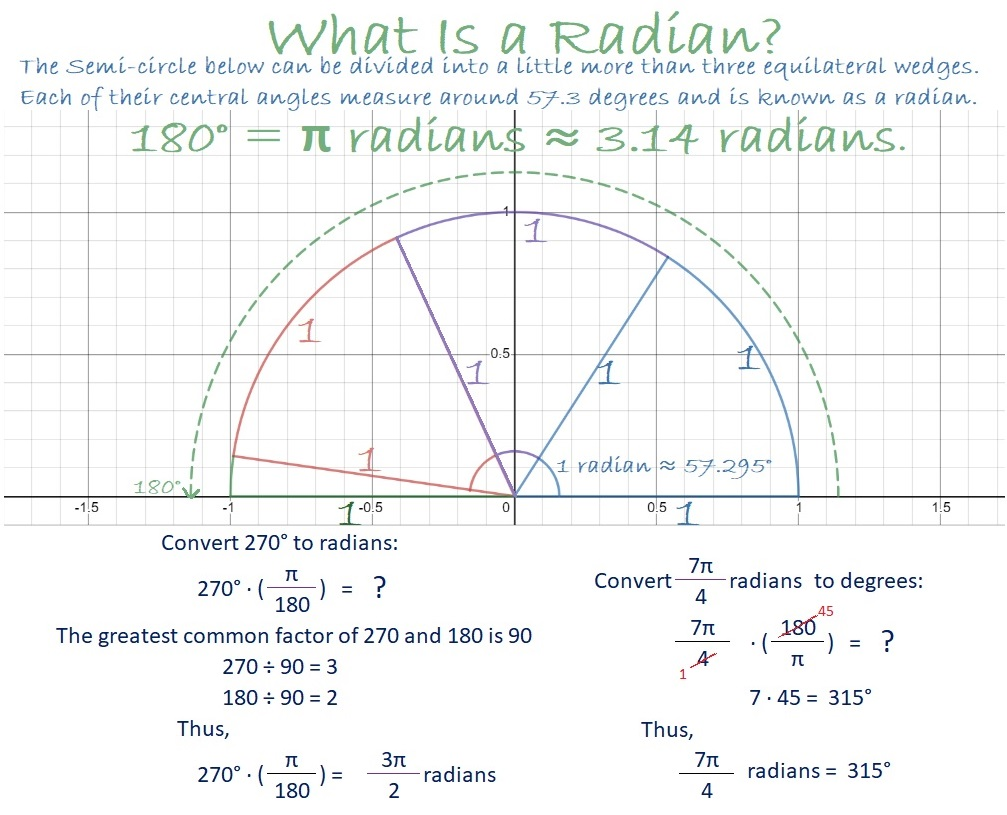Since I have students who rely on calculators but don’t have access to one during this pandemic, I designed a calculator in Desmos to convert Degrees to and from Radians. The calculator doesn’t work like magic, still requires you to think about what you are doing, and should prevent some of the common errors in doing such conversions.

### Factors of 1465:

• 1465 is a composite number.
• Prime factorization: 1465 = 5 × 293
• 1465 has no exponents greater than 1 in its prime factorization, so √1465 cannot be simplified.
• The exponents in the prime factorization are 1, and 1. Adding one to each exponent and multiplying we get (1 + 1)(1 + 1) = 2 × 2 = 4. Therefore 1465 has exactly 4 factors.
• The factors of 1465 are outlined with their factor pair partners in the graphic below.### More Facts about the Number 1465:

1465 is the sum of two squares in two different ways:
36² + 13² = 1465
32² + 21² = 1465

1465 is also the hypotenuse of FOUR Pythagorean triples:
340-1425-1465 which is 5 times 68-285-293.
583-1344-1465 calculated from 36² – 13², 2(36)(13), 36² + 13².
879-1172-1465 which is (3-4-5) times 293.
936-1127-1465 calculated from 2(36)(13), 36² – 13², 36² + 13².

From Stetson.edu, we learn this fun square number fact:
1465² = 2146225.

# 1463 When to Use the Law of Sines and the Law of Cosines

For years I have taught my students the law of sines and the law of cosines using only a hand-held scientific calculator. Unfortunately, many of them don’t have access to one during the pandemic. I found some online law of sines and law of cosines calculators, but I felt like they all seemed to find solutions by magic, without requiring any understanding. I want my students to understand when to use the law of sines and when to use the law of cosines.

### When Should I use the Law of Sines?

Use the law of sines when you are given these three pieces of information about a triangle: Angle-Angle-Side, Angle-Side-Angle, or Angle-Side-Side. Here’s a graphic I made showing each case and how the law of sines is transformed to obtain the missing angle or side: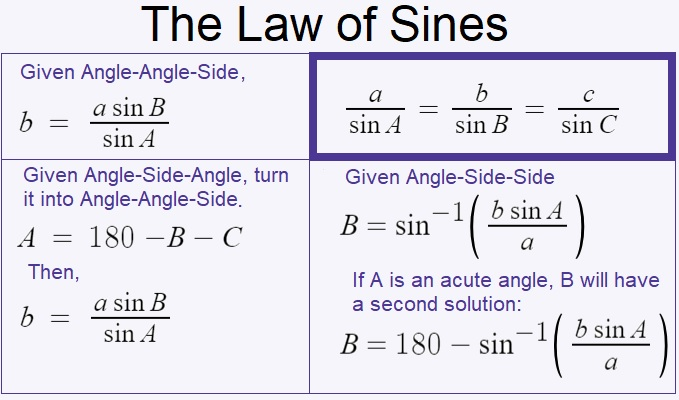I used Desmos to make a Law of Sines calculator that uses the above equations derived from the law of sines. Angles will be entered and given in degrees, not radians. This Law of Sines calculator still forces you to think about what information you’re given and which equation you should use. In your problem, there will be a known angle and a known side that are opposite to each other. Label these known values A and a, respectively. If you have another angle, label it B. If you have another side, label it b. Find the appropriate equation in the calculator, replace the variables with their values, and Desmos will calculate the answer. If you’re given Angle-Side-Side, you will need to use the inverse sine function, and you might have two solutions. Thus, if the given angle is acute, be sure to replace “Bacute” with the acute angle found in the formula above it so that you will also have that second solution. A triangle can’t have two obtuse angles, so don’t give two solutions for B if A is obtuse!

Some people might try to add sliders to that calculator because then they won’t have to think as much. Adding sliders would confuse Desmos with too many variables, so I also made a law of sines calculator with sliders. Angles will be entered and given in degrees, not radians. In your problem, there will be a known angle and a known side that are opposite to each other. Label these known values A and a, respectively. If you have another angle, label it B. If you have another side, label it b. Instead of typing the values into the equation, you can type the values in as sliders. The default for all the sliders is set at 0, so if any of your solutions is 0 or undefined, you are either looking in the wrong place for your answer or you haven’t entered enough information. If you use that calculator, you will need to refresh and reload it after each problem.

### When Should I use the Law of Cosines?

The Law of Cosines is easy enough to type: c² = a² + b² – 2ab cos C. It looks a little like the Pythagorean Theorem, and when C is 90º, that’s exactly what it is.

Use the law of cosines when you are given these three pieces of information about a triangle: Side-Angle-Side or Side-Side-Side. Here’s a graphic I made showing both cases:Since the Law of Cosines requires both a and b to be entered twice, I decided to go ahead and use sliders in the Law of Cosines calculator to make typing a little easier. Angles will be entered and given in degrees, not radians. If you are given Side-Angle-Side, let the angle be C. If you are given Side-Side-Side, label the side opposite the angle you are looking for, c. It doesn’t matter which side you label a or b, but that opposite side must be c. You will need to use the inverse cosine function, but I already have that in the calculator for you. You will need to refresh and reload before starting a new problem.

Since this is post number 1463, I’ll write a little bit about that number.

### Factors of 1463:

Since both 14 and 63 are multiples of 7, we can be sure that 1463 is divisible by 7.

• 1463 is a composite number.
• Prime factorization: 1463 = 7 × 11 × 19
• 1463 has no exponents greater than 1 in its prime factorization, so √1463 cannot be simplified.
• The exponents in the prime factorization are 1, 1, and 1. Adding one to each exponent and multiplying we get (1 + 1)(1 + 1)(1 + 1) = 2 × 2 × 2 = 8. Therefore 1463 has exactly 8 factors.
• The factors of 1463 are outlined with their factor pair partners in the graphic below.Since all four of the factor pairs of 1463 contain only odd numbers, 1463 can be written as the difference of two squares based on those four ways:

732² – 731² = 1463 (732 is the average of 1 and 1463, and 731 is one less.)
108² – 101² = 1463 (108 is the average of 7 and 209, and 101 is seven less.)
72² – 61² = 1463 (72 is the average of 11 and 133, and 61 is eleven less.)
48² – 29² = 1463 (48 is the average of 19 and 77, and 29 is nineteen less.)

# 1462 Has a Palindromic Factor Pair

There are only forty-five numbers between 100 and 10,000 that have palindromic factor pairs. That’s really not very many.

1462 is one of those numbers. That is pretty amazing, all by itself, but 1462 is also only four numbers more than another number on the list. That’s even more amazing!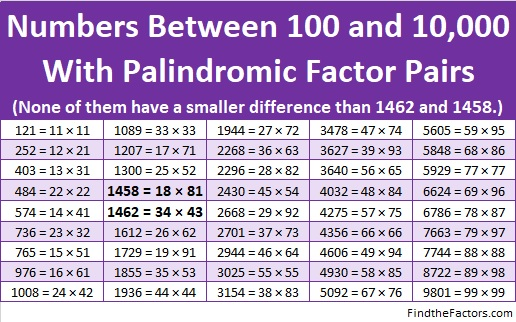Here are some more facts about the number 1462:

• 1462 is a composite number.
• Prime factorization: 1462 = 2 × 17 × 43
• 1462 has no exponents greater than 1 in its prime factorization, so √1462 cannot be simplified.
• The exponents in the prime factorization are 1, 1, and 1. Adding one to each exponent and multiplying we get (1 + 1)(1 + 1)(1 + 1) = 2 × 2 × 2 = 8. Therefore 1462 has exactly 8 factors.
• The factors of 1462 are outlined with their factor pair partners in the graphic below.1462 is the hypotenuse of one Pythagorean triple:
688-1290-1462 which is (8-15-17) times 86

# 1457 and Level 3

### Today’s Puzzle:

What numbers are common factors of 35 and 30? That’s the first question you need to solve this puzzle. All that’s left afterward is to look at the clues in the puzzle from top to bottom and write their factors in the factor column and the factor row. Remember, only use numbers from 1 to 10 as factors.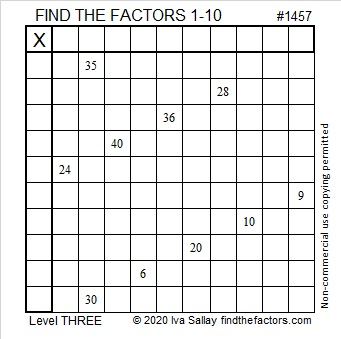### Factors of 1457:

• 1457 is a composite number.
• Prime factorization: 1457 = 31 × 47
• 1457 has no exponents greater than 1 in its prime factorization, so √1457 cannot be simplified.
• The exponents in the prime factorization are 1, and 1. Adding one to each exponent and multiplying we get (1 + 1)(1 + 1) = 2 × 2 = 4. Therefore 1457 has exactly 4 factors.
• The factors of 1457 are outlined with their factor pair partners in the graphic below.### Facts about the number 1457:

1457 is the difference of two squares in two different ways:
729² – 728² = 1457
39² – 8² = 1457

# 1456 and Level 2

#### Today’s Puzzle:

Can you write each number from 1 to 10 in both the first column and the top row so that those numbers are the factors of the given clues?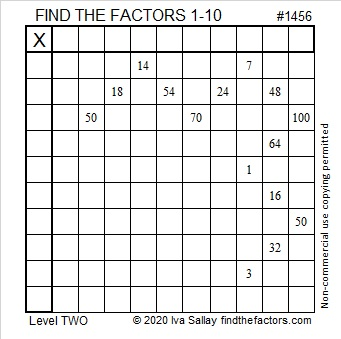#### 1456 Factor Tree:

1456 is made from two multiples of 7, so we know it is divisible by 7. It’s last two digits are 56, so it is divisible by 4. Since it is divisible by 7 and by 4, it is divisible by 28. I used that fact to make this factor tree for 1456: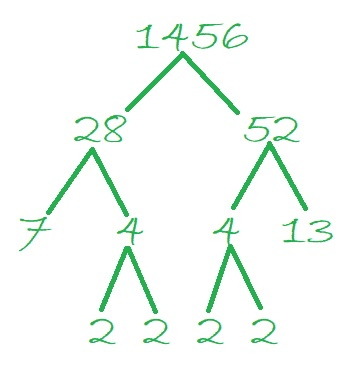#### Factors of 1456:

• 1456 is a composite number.
• Prime factorization: 1456 = 2 × 2 × 2 × 2 × 7 × 13, which can be written 1456 = 2⁴ × 7 × 13
• 1456 has at least one exponent greater than 1 in its prime factorization so √1456 can be simplified. Taking the factor pair from the factor pair table below with the largest square number factor, we get √1456 = (√16)(√91) = 4√91. The exponents in the prime factorization are 4, 1, and 1. Adding one to each exponent and multiplying we get (4 + 1)(1 + 1)(1 + 1) = 5 × 2 × 2 = 20. Therefore 1456 has exactly 20 factors.
• The factors of 1456 are outlined with their factor pair partners in the graphic below:#### Interesting facts about the number 1456:

1456 is the difference of two squares in six different ways:
365² – 363² = 1456
184² – 180² = 1456
95² – 87² = 1456
59² – 45² = 1456
41² – 15² = 1456
40² – 12² = 1456

1456 is the hypotenuse of one Pythagorean triple:
560-1344-1456 which is (5-12-13) times 112

# 135th Playful Math Education Blog CarnivalLadies and gentlemen welcome to the Playful Math Education Blog Carnival! This month’s carnival features the versatile number 135. It is the smallest number whose digits are the first three odd numbers. Watch 135 perform these AMAZING stunts:
1351 x 3³ 5¹
135 = (1+3+5)(1×3×5)
135 = 1¹ + 3² +5³
135 made that last one look as easy as 1-2-3.

### Carnival Wait Times

Now before we get started with our playful math blog posts, I would like to address wait times. Every worthwhile amusement park and carnival has lines in which people must wait. The playful math carnival loves lines and is no exception, but is waiting really a bad thing? In Solving 100÷3 Mentally: a Surprise, Marilyn Burns teaches and assesses children’s understanding of mathematics. She explains that patiently waiting for kids’ mathematical thoughts can actually speed up their understanding and enjoyment. Hence, it is all worth the wait!

Mr. Mathematics also waits patiently for his students to think problems through themselves. When an inspector tried to interview him about issues he faces in his department, he turned the tables and talked about his students’ learning so much because he waits patiently for his students to problem solve.

### Art

Robert Loves Pi has makes gorgeous mathematical art. For example, he’s made this design with circles, triangles and a pentagon and a beautiful rotating arrangement of a pentagrammic prism.

Since it is February, Colleen Young collected a very nice assortment of hearts related to mathematical content.

Mike and his sons of Mike’s Math Page produced some lovely geometric designs in A Fun Zometool project with Decagons.

Paula Beardell Krieg has published several blog posts on Frieze symmetry. Here is the start of that series of posts:

### Education

I’ve seen the visual below several times before, but I didn’t realize its magnificence until I read Sara VanDerWerf post describing how she has used it to inspire herself, her students, and other teachers:

Ben Orlin has tips from four math teachers on what makes a teacher great:

### Food Court at the Math Carnival

Here’s a tasty blog post:

### Geometry

Did you know that 81² + 108² = 135²? That’s simply 27 times 3² + 4²= 5², the most famous example of the Pythagorean Theorem. The scarecrow from the Wizard of Oz sounds impressive when he recites something that sounds a little like the Pythagorean Theorem. Is the formula he gives true? Watch this short movie clip and tell me what you think:

Check out these geometry blog posts I saw on twitter:

### Language Arts and Math

If you just asked students to write something about math, they might not have much to say, but if you gave them some of the wonderful prompts suggested by CLopen Mathdebater in Explore Math with Prompts, you might just be as pleasantly surprised as she was with her student’s writing and mathematical thinking!

Intersections–Poetry with Mathematics writes about a particular subject we’ve all seen in both word problems and nightmares: Those Trains in Word Problems–Who Rides Them?

Kelly Darke of Math Book Magic has been reading picture books about counting with her child. She explains why they found the picture book, 1-2-3 Peas, to be magical. She loved watching her child trace the illustrated numerals as they explored bigger numbers like 80 and made connections between numerals and the letters of the alphabet.

### Math News Room

Here are some news articles related to math that I enjoyed reading this month:

### Museum of Math

Pat’s Blog can tell you what mathematical event happened on today’s date. For example, here is mathematical history for February 10.

Life Through a Mathematician’s Eyes shares the highlights of a history of mathematics for January.

The following images would fit in perfectly in any math museum:

### Probability and Statistics

Fraction Fanatic has been sharing resources every week since the beginning of 2020. In the first post of the year, This week, Number 1, we see ways to make leaf and stem plots and to make predicting probability both pertinent and fun.

Joseph Nebus regularly shares mathematically themed comics on his blog. In this one, he shares a comic that points out that those probabilities pertain to you personally, not just everybody else.

### Puzzles

Mathtuition shares a math puzzle that will get kids in primary school thinking in Marbles Math Question.

Here are some other mathematical puzzles from blogs that I saw on twitter:

### Strategies

K-8 Math Specialist Jenna Leib writes about learning and having fun with ten frames and tiny polka dots in Kindergarten Debate:  Building Appreciation for Ten Frames. By the way, it’s the kindergarteners who are debating, not adults!

Given two ordered pairs, how do you find their midpoint? Don’t use some cheap trick. Math Chat has a midpoint strategy that students will remember forever, and it promotes mathematical understanding.

The writer behind Math QED earned a 770 on the math portion of the SAT and lists strategies to help you get a perfect score on that part of the test as well.

Here are a couple more blog posts about strategies that were shared on twitter:

### More Carnivals

I hope you enjoyed all the attractions at this month’s Playful Math Education Blog Carnival.

Last month the carnival was hosted by Math Misery?, but so far no one has volunteered to host the carnival for the end of March. Perhaps you will consider hosting it! It is a lot of fun exploring other people’s blogs and selecting what to share, so do think about hosting it next month or some other time in the future. Click here to volunteer to host or to submit one of your posts to the carnival.

You may also want to check out February’s Carnival of Mathematics hosted by Stormy at Storm Bear World.

# 1454 Happy Valentines’ Day

I hope that today and always you have lots of love in your heart and that you feel so much love from others. Have a wonderful Valentine’s Day!

#### A Valentines Puzzle

Here is a Valentines themed mystery level puzzle from me to you:#### Factors of 1454

• 1454 is a composite number.
• Prime factorization: 1454 = 2 × 727
• 1454 has no exponents greater than 1 in its prime factorization, so √1454 cannot be simplified.
• The exponents in the prime factorization are 1, and 1. Adding one to each exponent and multiplying we get (1 + 1)(1 + 1) = 2 × 2 = 4. Therefore 1454 has exactly 4 factors.
• The factors of 1454 are outlined with their factor pair partners in the graphic below.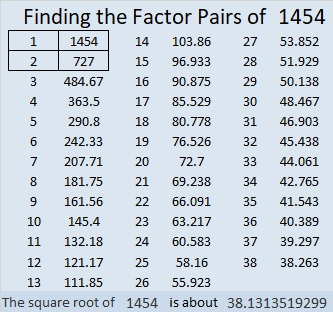#### Interesting Fact about the Number 1454:

From Stetson.edu we learn that 11+444+555+444=1454.

#### Mathematical Valentine Tweets I’ve Seen Today:

I hope those tweets help fuel your love for mathematics. Have a very happy Valentines’ Day!